Next: Surface Brightness Profiles Up: Photometric and Surface Brightness Previous: Introduction

# Stellar Photometry

In order to obtain a quantitative estimate of the accuracy and reliability of our data reduction procedures and results, we have performed a data reduction pipeline based on the following steps: i) define a set of simulated HST-like images with synthetic globular cluster stellar distributions, which provide the ``true'' data for comparison; ii) convolve these images with appropriate assumptions of PSF and noise in order to construct simulated ``observed'' data; iii) deconvolve the ``observed'' images using some of the most widely adopted techniques and assumptions; iv) reduce the ``deconvolved'' frames in a totally independent way, and compare with the initial ``true'' data. Specifically, for steps i) and ii) we used the set of HST-simulated images prepared by Hanisch (1993) (namely the files in the directory star_cluster), in order to be able to compare our results also with the work of other researchers in the field, while steps iii) and iv) were performed by two different teams of our group who ignored the assumptions made by the other team in order to assure the complete independence of each step. Since this work was started in September 1993, we adopted the version of Hanisch files then available, which is different from the revised version released on October 6, 1993. The characteristics of our files are then: (i) intensity-normalized PSF peak; (ii) stars centered on the pixels; and (iii) zero-point = 9.0 mag.

In summary, using a special version of the package ROMAFOT (Buonanno et al. 1983, Buonanno &Iannicola 1988), we have then reduced the following set of files:

a)
``Truth'': input star cluster with a globular cluster-like luminosity function (LF) and spatial distribution (Hanisch 1993). It contains 470 stars.

b)
``Observed'': HST-simulated ``star_cluster'' (Hanisch 1993) with 470 stars, old WFC (sim2.fit), new WF2 (sim2c.fit), size = 256
256, space invariant PSFs (psf12.fit, psfc12.fit), filter F555W, center of image, where ``sim2'' stands for the HST images uncorrected for spherical aberration, and ``sim2c'' are the HST-corrected frames, and hereafter label the two separate families of adopted simulations.

c)
``R-L deconvolved'': deconvolved with the Richardson and Lucy method (R-L) (Richardson 1972, Lucy 1974, 1992), using a variable number of accelerated iterations (50, 150, 250).

d)
``MEM deconvolved'': deconvolved with the Maximum Entropy Method (MEM), adopting two different versions, i.e., MEM-IDL (with 50, 150, 250 iterations; this routine was written by F. Varosi based on Hollis et al. 1992) and MEM/MemSys5 (with ICF = 0, 1; Weir 1991).

e)
``Wiener'': deconvolved with a Wiener-based routine (IRAF, package restore; Busko 1993).

In the reduction of all frames the star detection threshold has been set always at 5, the value calculated on the background empirically determined in the ``observed'' frames (i.e., 8 counts). Note that, due to the combination of the systematic centering of the stars in the pixel centers and the crowding effects, only 373 stars are actually detectable as independent objects on the ``observed'' frame listed in Table 1. The second column, labelled ``Detected'', contains the total number of identified peaks, including those which could not be fitted by the ROMAFOT stellar profile fitting routine and are interpreted as noise. Finally, note also that the two original ``observed'' images (Hanisch 1993) were PSF peak-intensity normalized, therefore the data obtained from the two families (Table 1 and Table 2) are not directly comparable as the corresponding stars have actually different S/N values.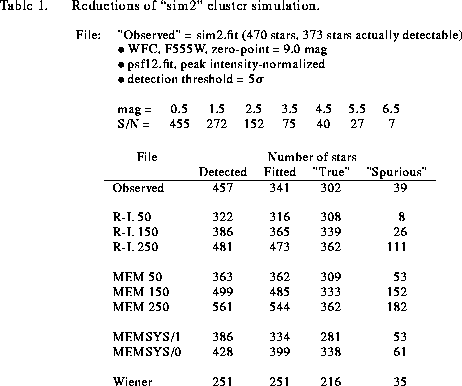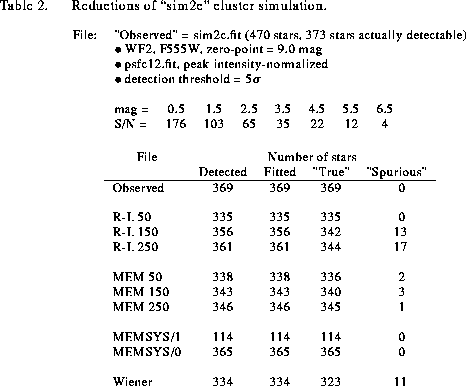Tables 1 and 2 and Figs. 1 and 2 report the summary of the results for the uncorrected and corrected images ``sim2''and ``sim2c'', respectively.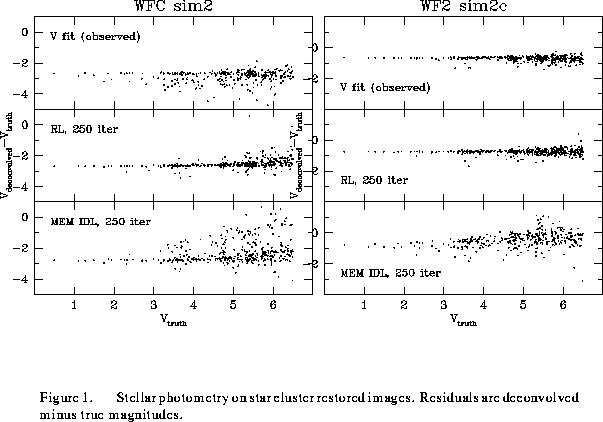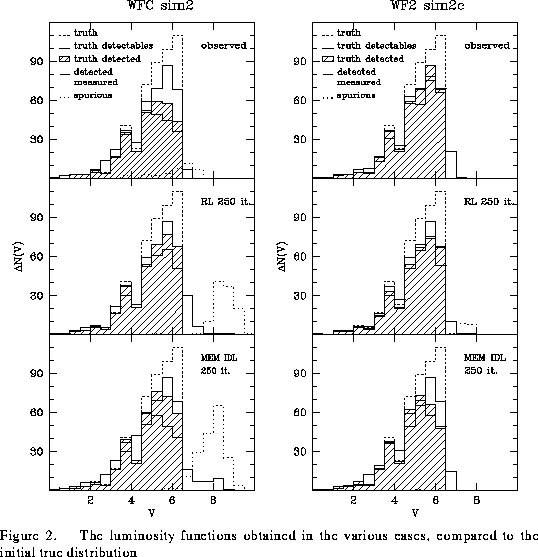The main conclusions one can draw from the tables and figures are:

1. With ``sim2'' (uncorrected HST), the number of detected ``true'' stars in the ``observed'' frame is about 20%smaller than the number of stars that could in principle be detected (302 vs. 373), and the number of ``fitted'' stars includes about 10%of spurious identifications. Overall, the completeness factor (i.e., ``true''/470) is about 65%, and it would presumably be somewhat larger if the stars were not assumed to be centered in the pixels (further tests are in progress using a revised version of the simulation where the stars are not centered in the pixel matrix). These numbers increase in the deconvolved images and with increasing the number of iterations, however, the number of ``spurious'' objects also increases steeply, correspondingly. The behavior of these quantities as a function of magnitude can be seen in Fig. 1.

2. With ``sim2c'' (corrected HST), the number of stars detected in the ``observed'' frame (before deconvolution) is almost coincident with the maximum attainable (369 vs. 373). The search on the deconvolved images (at a fixed threshold of 8 counts, as derived from the ``observed'' frame) yields a smaller number of detected objects. The stars lost are faint objects with peak intensities just over the threshold in the ``observed'' frame, which fall just below the threshold after deconvolution. This is due to the fact that all deconvolution methods tend to enhance the contrast, namely to increase slightly the peak intensity of the brightest stars at the expense of the weakest peaks which are damped as noise. Finally, it is remarkable to note the difference in number of detected objects obtained adopting ICF = 1 or 0 with MEMSYS.

3. The impact of these effects on the LFs consists in a ``deformation'' of the differential LF, with a shift of some objects to the adjacent brighter bin and a lack of stars close to the detection limit. The detection limit is of course defined by the adopted threshold, which was selected in order to optimize the number of detected ``true'' stars with respect to the spurious identifications.

4. Concerning the linearity, the internal photometric accuracy, and the zero-point checks, additional tests are in progress. In general, the photometry of safely uncrowded stars yields magnitudes accurate to better than 0.1 mag, including any systematic zero-point shift. In particular, the use of a Gaussian fit to the PSF plus a look-up table to map the systematic residuals already yields satisfactory results.Next: Surface Brightness Profiles Up: Photometric and Surface Brightness Previous: Introduction

rlw@sundog.stsci.edu
Fri Apr 15 17:35:57 EDT 1994